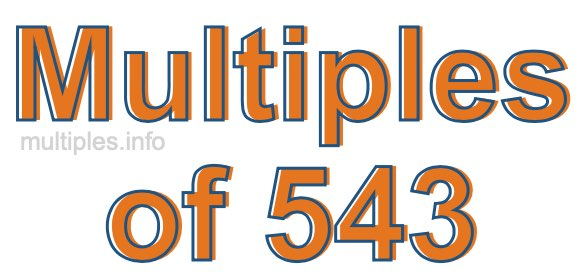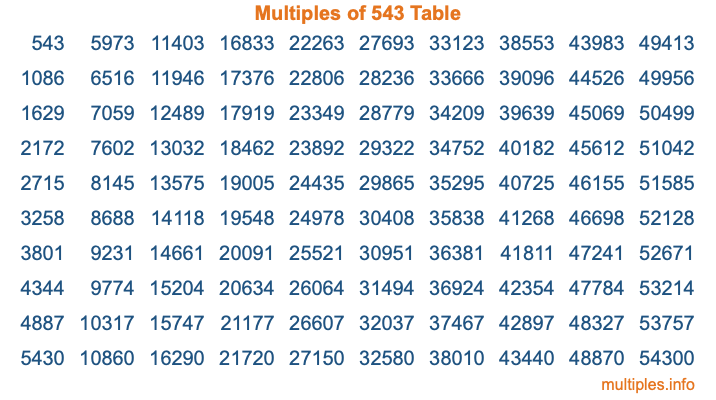Multiples of 543Welcome to the Multiples of 543 page. Here we will first teach you everything you will ever need to know about the multiples of 543, and then give you a study guide summary of everything we taught you to make sure you remember it all. Use this page to look up facts and learn information about the multiples of 543. This page will make you a multiples of five hundred forty-three expert!

Definition of Multiples of 543
Multiples of 543 are all the numbers that when divided by 543 equal an integer. Each of the multiples of 543 are called a multiple. A multiple of 543 is created by multiplying 543 by an integer.

Therefore, to create a list of multiples of 543, you start with 1 multiplied by 543, then 2 multiplied by 543, then 3 multiplied by 543, and so on for as long as you want. Thus, the list of the first five multiples of 543 is 543, 1086, 1629, 2172, and 2715. To see a larger list of multiples of 543, see the printable image of Multiples of 543 further down on this page. We also have a category where you can choose any nth multiple of 543.

Multiples of 543 Checker
The Multiples of 543 Checker below checks to see if any number of your choice is a multiple of 543. In other words, it checks to see if there is any number (integer) that when multiplied by 543 will equal your number. To do that, we divide your number by 543. If the the quotient is an integer, then your number is a multiple of 543.

Is  a multiple of 543?

Least Common Multiple of 543 and ...
A Least Common Multiple (LCM) is the lowest multiple that two or more numbers have in common. This is also called the smallest common multiple or lowest common multiple and is useful to know when you are adding our subtracting fractions. Enter one or more numbers below (543 is already entered) to find the LCM.

Check out our LCM Calculator if you need more details about the Least Common Multiple or if you need the LCM for different numbers for adding and subtraction fractions.

nth Multiple of 543
As we stated above, 543 is the first multiple of 543, 1086 is the second multiple of 543, 1629 is the third multiple of 543, and so on. Enter a number below to find the nth multiple of 543.

th multiple of 543

Multiples of 543 vs Factors of 543
543 is a multiple of 543 and a factor of 543, but that is where the similarities end. All postive multiples of 543 are 543 or greater than 543. All positive factors of 543 are 543 or less than 543.

Below is the beginning list of multiples of 543 and the factors of 543 so you can compare:

Multiples of 543: 543, 1086, 1629, 2172, 2715, etc.

Factors of 543: 1, 3, 181, 543

As you can see, the multiples of 543 are all the numbers that you can divide by 543 to get a whole number. The factors of 543, on the other hand, are all the whole numbers that you can multiply by another whole number to get 543.

It's also interesting to note that if a number (x) is a factor of 543, then 543 will also be a multiple of that number (x).

Multiples of 543 vs Divisors of 543
The divisors of 543 are all the integers that 543 can be divided by evenly. Below is a list of the divisors of 543.

Divisors of 543: 1, 3, 181, 543

The interesting thing to note here is that if you take any multiple of 543 and divide it by a divisor of 543, you will see that the quotient is an integer.

Multiples of 543 Table
Below is an image of the first 100 multiples of 543 in a table. The table is in chronological order, column by column. The first column has the first ten multiples of 543, the second column has the next ten multiples of 543, and so on.The Multiples of 543 Table is also referred to as the 543 Times Table or Times Table of 543. You are welcome to print out our table for your studies.

Negative Multiples of 543
Although not often discussed or needed in math, it is worth mentioning that you can make a list of negative multiples of 543 by multiplying 543 by -1, then by -2, then by -3, and so on, to get the following list of negative multiples of 543:

-543, -1086, -1629, -2172, -2715, etc.

Multiples of 543 Summary
Below is a summary of important Multiples of 543 facts that we have discussed on this page. To retain the knowledge on this page, we recommend that you read through the summary and explain to yourself or a study partner why they hold true.

There are an infinite number of multiples of 543.

A multiple of 543 divided by 543 will equal a whole number.

543 divided by a factor of 543 equals a divisor of 543.

The nth multiple of 543 is n times 543.

The largest factor of 543 is equal to the first positive multiple of 543.

543 is a multiple of every factor of 543.

543 is a multiple of 543.

A multiple of 543 divided by a divisor of 543 equals an integer.

543 divided by a divisor of 543 equals a factor of 543.

Any integer times 543 will equal a multiple of 543.

Multiples of a Number
Here you can get the multiples of another number, all with the same attention to detail as we did for multiples of 543 on this page.

Multiples of
Multiples of 544
Did you find our page about multiples of five hundred forty-three educational? Do you want more knowledge? Check out the multiples of the next number on our list!